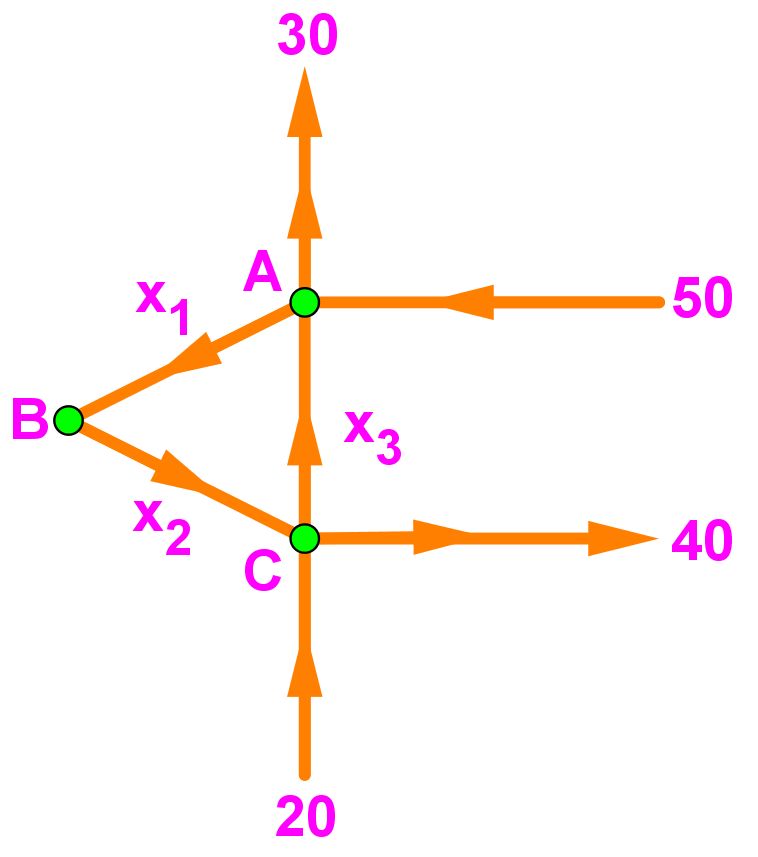# Applications of linear systems

### Applications of linear systems

#### Lessons

We will be looking at real-life problems involving linear algebra. The three types of real-life applications we will be looking at are:
1. Linear systems in Economics
2. Linear systems with Chemical Equations
3. Linear systems with Network Flow

In economics, we can use linear algebra to determine the equilibrium price of outputs for each sector. Note that in order to get the equilibrium price, we need to set

Income = expenses (expenditures)

In Chemistry, we can use linear algebra to balance chemical equations like:
$N_2+H_2$$NH_3$

We do so by counting the number of elements in a compound, and turning each coefficient as a variable to solve.

We can also use linear algebra to study the flow of some quantity through a network. Make sure that for each node:
Flow in = Flow out

Our goal is to make all of these questions into matrix, and then solve.
• Introduction
Applications of Linear Systems Overview:
a)
Linear Systems in Economics
• A simple economy with sectors.
• Solving the equilibrium prices

b)
Linear Systems with Chemical Equations
• Balancing chemical equations
• Solving for coefficients to balance

c)
Linear Systems with Network Flow
• Nodes
• Finding the general solution of the network flow

• 1.
Economics with Resources
Assume that the economy has 3 sectors: Manufacturing, Services, and Extraction. Manufacturing sells 50% of its output to Services, and 50% to Extraction. Services sells 30% of its output to Manufacturing, 60% to extraction, and keeps the rest. Extraction sells 80% of its output to manufacturing, 10% to services, and keeps the rest. Find a set of equilibrium prices for when the extraction output is 80 units.

• 2.
Balancing a Chemical Equation
Balance the following chemical equation using the vector equations
$Na_3 PO_4+MgCl_2$$NaCl+Mg_3(PO_4)_2$

• 3.
Finding the General Flow Pattern
Find the general solution of the network flow. Assuming that all flows are non-negative, what is the minimum value of $x_1$ and $x_2$?